# fitrgp

Fit a Gaussian process regression (GPR) model

## Syntax

``gprMdl = fitrgp(Tbl,ResponseVarName)``
``gprMdl = fitrgp(Tbl,formula)``
``gprMdl = fitrgp(Tbl,y)``
``gprMdl = fitrgp(X,y)``
``gprMdl = fitrgp(___,Name,Value)``

## Description

example

``gprMdl = fitrgp(Tbl,ResponseVarName)` returns a Gaussian process regression (GPR) model trained using the sample data in `Tbl`, where `ResponseVarName` is the name of the response variable in `Tbl`.`
``gprMdl = fitrgp(Tbl,formula)` returns a Gaussian process regression (GPR) model, trained using the sample data in `Tbl`, for the predictor variables and response variables identified by `formula`.`
``gprMdl = fitrgp(Tbl,y)` returns a GPR model for the predictors in table `Tbl` and continuous response vector `y`.`

example

``gprMdl = fitrgp(X,y)` returns a GPR model for predictors `X` and continuous response vector `y`.`

example

````gprMdl = fitrgp(___,Name,Value)` returns a GPR model for any of the input arguments in the previous syntaxes, with additional options specified by one or more `Name,Value` pair arguments.For example, you can specify the fitting method, the prediction method, the covariance function, or the active set selection method. You can also train a cross-validated model.`gprMdl` is a `RegressionGP` object. For object functions and properties of this object, see `RegressionGP`.If you train a cross-validated model, then `gprMdl` is a `RegressionPartitionedGP` object. For further analysis on the cross-validated object, use the object functions of the `RegressionPartitionedGP` object.```

## Examples

collapse all

This example uses the abalone data , , from the UCI Machine Learning Repository  . Download the data and save it in your current folder with the name `abalone.data`.

Store the data into a table. Display the first seven rows.

```tbl = readtable('abalone.data','Filetype','text',... 'ReadVariableNames',false); tbl.Properties.VariableNames = {'Sex','Length','Diameter','Height',... 'WWeight','SWeight','VWeight','ShWeight','NoShellRings'}; tbl(1:7,:)```
```ans = Sex Length Diameter Height WWeight SWeight VWeight ShWeight NoShellRings ___ ______ ________ ______ _______ _______ _______ ________ ____________ 'M' 0.455 0.365 0.095 0.514 0.2245 0.101 0.15 15 'M' 0.35 0.265 0.09 0.2255 0.0995 0.0485 0.07 7 'F' 0.53 0.42 0.135 0.677 0.2565 0.1415 0.21 9 'M' 0.44 0.365 0.125 0.516 0.2155 0.114 0.155 10 'I' 0.33 0.255 0.08 0.205 0.0895 0.0395 0.055 7 'I' 0.425 0.3 0.095 0.3515 0.141 0.0775 0.12 8 'F' 0.53 0.415 0.15 0.7775 0.237 0.1415 0.33 20```

The dataset has 4177 observations. The goal is to predict the age of abalone from eight physical measurements. The last variable, number of shell rings shows the age of the abalone. The first predictor is a categorical variable. The last variable in the table is the response variable.

Fit a GPR model using the subset of regressors method for parameter estimation and fully independent conditional method for prediction. Standardize the predictors.

```gprMdl = fitrgp(tbl,'NoShellRings','KernelFunction','ardsquaredexponential',... 'FitMethod','sr','PredictMethod','fic','Standardize',1) ```
```grMdl = RegressionGP PredictorNames: {1x8 cell} ResponseName: 'Var9' ResponseTransform: 'none' NumObservations: 4177 KernelFunction: 'ARDSquaredExponential' KernelInformation: [1x1 struct] BasisFunction: 'Constant' Beta: 10.9148 Sigma: 2.0243 PredictorLocation: [10x1 double] PredictorScale: [10x1 double] Alpha: [1000x1 double] ActiveSetVectors: [1000x10 double] PredictMethod: 'FIC' ActiveSetSize: 1000 FitMethod: 'SR' ActiveSetMethod: 'Random' IsActiveSetVector: [4177x1 logical] LogLikelihood: -9.0013e+03 ActiveSetHistory: [1x1 struct] BCDInformation: [] ```

Predict the responses using the trained model.

`ypred = resubPredict(gprMdl);`

Plot the true response and the predicted responses.

```figure(); plot(tbl.NoShellRings,'r.'); hold on plot(ypred,'b'); xlabel('x'); ylabel('y'); legend({'data','predictions'},'Location','Best'); axis([0 4300 0 30]); hold off;```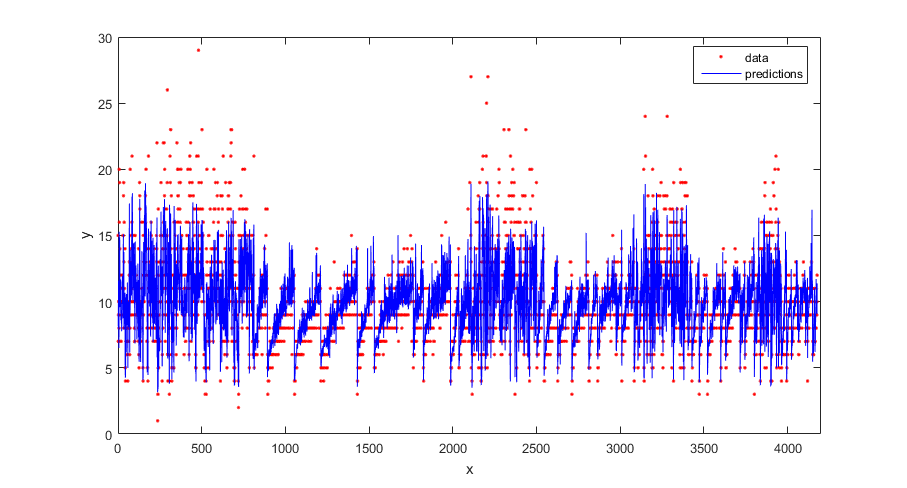Compute the regression loss on the training data (resubstitution loss) for the trained model.

`L = resubLoss(gprMdl)`
```L = 4.0064```

Generate sample data.

```rng(0,'twister'); % For reproducibility n = 1000; x = linspace(-10,10,n)'; y = 1 + x*5e-2 + sin(x)./x + 0.2*randn(n,1);```

Fit a GPR model using a linear basis function and the exact fitting method to estimate the parameters. Also use the exact prediction method.

```gprMdl = fitrgp(x,y,'Basis','linear',... 'FitMethod','exact','PredictMethod','exact');```

Predict the response corresponding to the rows of `x` (resubstitution predictions) using the trained model.

`ypred = resubPredict(gprMdl);`

Plot the true response with the predicted values.

```plot(x,y,'b.'); hold on; plot(x,ypred,'r','LineWidth',1.5); xlabel('x'); ylabel('y'); legend('Data','GPR predictions'); hold off```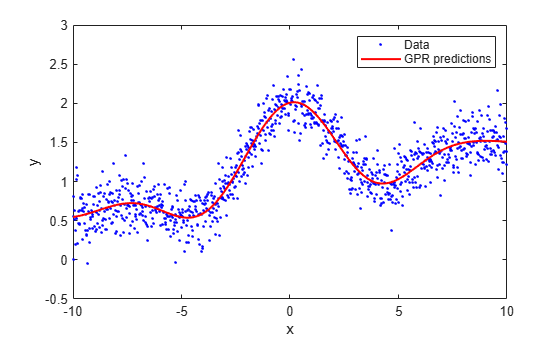`load('gprdata2.mat')`

The data has one predictor variable and continuous response. This is simulated data.

Fit a GPR model using the squared exponential kernel function with default kernel parameters.

`gprMdl1 = fitrgp(x,y,'KernelFunction','squaredexponential');`

Now, fit a second model, where you specify the initial values for the kernel parameters.

```sigma0 = 0.2; kparams0 = [3.5, 6.2]; gprMdl2 = fitrgp(x,y,'KernelFunction','squaredexponential',... 'KernelParameters',kparams0,'Sigma',sigma0);```

Compute the resubstitution predictions from both models.

```ypred1 = resubPredict(gprMdl1); ypred2 = resubPredict(gprMdl2);```

Plot the response predictions from both models and the responses in training data.

```figure(); plot(x,y,'r.'); hold on plot(x,ypred1,'b'); plot(x,ypred2,'g'); xlabel('x'); ylabel('y'); legend({'data','default kernel parameters',... 'kparams0 = [3.5,6.2], sigma0 = 0.2'},... 'Location','Best'); title('Impact of initial kernel parameter values'); hold off```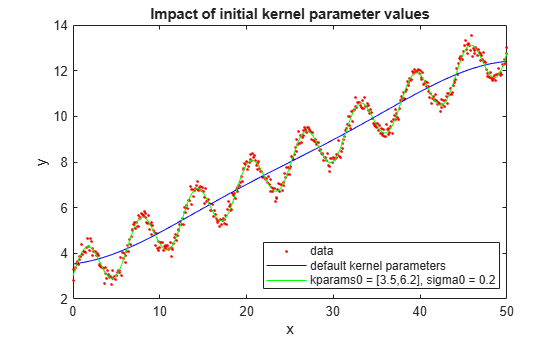The marginal log likelihood that `fitrgp` maximizes to estimate GPR parameters has multiple local solutions; the solution that it converges to depends on the initial point. Each local solution corresponds to a particular interpretation of the data. In this example, the solution with the default initial kernel parameters corresponds to a low frequency signal with high noise whereas the second solution with custom initial kernel parameters corresponds to a high frequency signal with low noise.

`load('gprdata.mat')`

There are six continuous predictor variables. There are 500 observations in the training data set and 100 observations in the test data set. This is simulated data.

Fit a GPR model using the squared exponential kernel function with a separate length scale for each predictor. This covariance function is defined as:

`$k\left({x}_{i},{x}_{j}|\theta \right)={\sigma }_{f}^{2}\phantom{\rule{0.05555555555555556em}{0ex}}\phantom{\rule{0.05555555555555556em}{0ex}}exp\left[-\frac{1}{2}\sum _{m=1}^{d}\frac{{\left({x}_{im}-{x}_{jm}\right)}^{2}}{{\sigma }_{m}^{2}}\right].$`

where ${\sigma }_{m}$ represents the length scale for predictor $m$, $m$ = 1, 2, ..., $d$ and ${\sigma }_{f}$ is the signal standard deviation. The unconstrained parametrization $\theta$ is

`$\begin{array}{l}{\theta }_{m}=\mathrm{log}{\sigma }_{m},\phantom{\rule{1em}{0ex}}for\phantom{\rule{0.2777777777777778em}{0ex}}m=1,2,...,d\phantom{\rule{1em}{0ex}}\\ {\theta }_{d+1}=\mathrm{log}{\sigma }_{f}.\end{array}$`

Initialize length scales of the kernel function at 10 and signal and noise standard deviations at the standard deviation of the response.

```sigma0 = std(ytrain); sigmaF0 = sigma0; d = size(Xtrain,2); sigmaM0 = 10*ones(d,1);```

Fit the GPR model using the initial kernel parameter values. Standardize the predictors in the training data. Use the exact fitting and prediction methods.

```gprMdl = fitrgp(Xtrain,ytrain,'Basis','constant','FitMethod','exact',... 'PredictMethod','exact','KernelFunction','ardsquaredexponential',... 'KernelParameters',[sigmaM0;sigmaF0],'Sigma',sigma0,'Standardize',1);```

Compute the regression loss on the test data.

`L = loss(gprMdl,Xtest,ytest)`
```L = 0.6919 ```

Access the kernel information.

`gprMdl.KernelInformation`
```ans = struct with fields: Name: 'ARDSquaredExponential' KernelParameters: [7x1 double] KernelParameterNames: {7x1 cell} ```

Display the kernel parameter names.

`gprMdl.KernelInformation.KernelParameterNames`
```ans = 7x1 cell {'LengthScale1'} {'LengthScale2'} {'LengthScale3'} {'LengthScale4'} {'LengthScale5'} {'LengthScale6'} {'SigmaF' } ```

Display the kernel parameters.

`sigmaM = gprMdl.KernelInformation.KernelParameters(1:end-1,1)`
```sigmaM = 6×1 104 × 0.0004 0.0007 0.0004 4.7665 0.1018 0.0056 ```
`sigmaF = gprMdl.KernelInformation.KernelParameters(end)`
```sigmaF = 28.1720 ```
`sigma = gprMdl.Sigma`
```sigma = 0.8162 ```

Plot the log of learned length scales.

```figure() plot((1:d)',log(sigmaM),'ro-'); xlabel('Length scale number'); ylabel('Log of length scale');```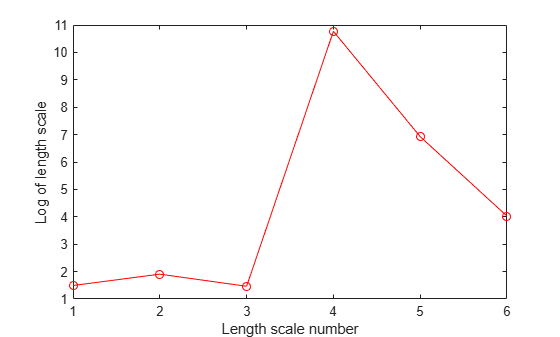The log of length scale for the 4th and 5th predictor variables are high relative to the others. These predictor variables do not seem to be as influential on the response as the other predictor variables.

Fit the GPR model without using the 4th and 5th variables as the predictor variables.

```X = [Xtrain(:,1:3) Xtrain(:,6)]; sigma0 = std(ytrain); sigmaF0 = sigma0; d = size(X,2); sigmaM0 = 10*ones(d,1); gprMdl = fitrgp(X,ytrain,'Basis','constant','FitMethod','exact',... 'PredictMethod','exact','KernelFunction','ardsquaredexponential',... 'KernelParameters',[sigmaM0;sigmaF0],'Sigma',sigma0,'Standardize',1);```

Compute the regression error on the test data.

```xtest = [Xtest(:,1:3) Xtest(:,6)]; L = loss(gprMdl,xtest,ytest)```
```L = 0.6928 ```

The loss is similar to the one when all variables are used as predictor variables.

Compute the predicted response for the test data.

` ypred = predict(gprMdl,xtest);`

Plot the original response along with the fitted values.

```figure; plot(ytest,'r'); hold on; plot(ypred,'b'); legend('True response','GPR predicted values','Location','Best'); hold off```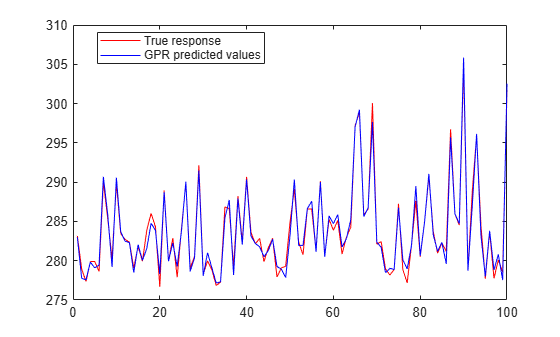This example shows how to optimize hyperparameters automatically using `fitrgp`. The example uses the `gprdata2` data that ships with your software.

`load('gprdata2.mat')`

The data has one predictor variable and continuous response. This is simulated data.

Fit a GPR model using the squared exponential kernel function with default kernel parameters.

`gprMdl1 = fitrgp(x,y,'KernelFunction','squaredexponential');`

Find hyperparameters that minimize five-fold cross-validation loss by using automatic hyperparameter optimization.

For reproducibility, set the random seed and use the `'expected-improvement-plus'` acquisition function.

```rng default gprMdl2 = fitrgp(x,y,'KernelFunction','squaredexponential',... 'OptimizeHyperparameters','auto','HyperparameterOptimizationOptions',... struct('AcquisitionFunctionName','expected-improvement-plus'));```
```|======================================================================================| | Iter | Eval | Objective: | Objective | BestSoFar | BestSoFar | Sigma | | | result | log(1+loss) | runtime | (observed) | (estim.) | | |======================================================================================| | 1 | Best | 0.29417 | 3.0183 | 0.29417 | 0.29417 | 0.0015045 | | 2 | Best | 0.037898 | 1.8402 | 0.037898 | 0.060792 | 0.14147 | | 3 | Accept | 1.5693 | 1.3938 | 0.037898 | 0.040633 | 25.279 | | 4 | Accept | 0.29417 | 4.5525 | 0.037898 | 0.037984 | 0.0001091 | | 5 | Accept | 0.29393 | 2.7292 | 0.037898 | 0.038029 | 0.029932 | | 6 | Accept | 0.13152 | 1.6443 | 0.037898 | 0.038127 | 0.37127 | | 7 | Best | 0.037785 | 1.7693 | 0.037785 | 0.037728 | 0.18116 | | 8 | Accept | 0.03783 | 1.7648 | 0.037785 | 0.036524 | 0.16251 | | 9 | Accept | 0.037833 | 1.6799 | 0.037785 | 0.036854 | 0.16159 | | 10 | Accept | 0.037835 | 1.662 | 0.037785 | 0.037052 | 0.16072 | | 11 | Accept | 0.29417 | 2.5872 | 0.037785 | 0.03705 | 0.00038214 | | 12 | Accept | 0.42256 | 1.2553 | 0.037785 | 0.03696 | 3.2067 | | 13 | Accept | 0.03786 | 1.944 | 0.037785 | 0.037087 | 0.15245 | | 14 | Accept | 0.29417 | 2.7661 | 0.037785 | 0.037043 | 0.0063584 | | 15 | Accept | 0.42302 | 2.6525 | 0.037785 | 0.03725 | 1.2221 | | 16 | Accept | 0.039486 | 1.9644 | 0.037785 | 0.037672 | 0.10069 | | 17 | Accept | 0.038591 | 3.0923 | 0.037785 | 0.037687 | 0.12077 | | 18 | Accept | 0.038513 | 2.9921 | 0.037785 | 0.037696 | 0.1227 | | 19 | Best | 0.037757 | 2.0477 | 0.037757 | 0.037572 | 0.19621 | | 20 | Accept | 0.037787 | 2.0042 | 0.037757 | 0.037601 | 0.18068 | |======================================================================================| | Iter | Eval | Objective: | Objective | BestSoFar | BestSoFar | Sigma | | | result | log(1+loss) | runtime | (observed) | (estim.) | | |======================================================================================| | 21 | Accept | 0.44917 | 1.1509 | 0.037757 | 0.03766 | 8.7818 | | 22 | Accept | 0.040201 | 1.5921 | 0.037757 | 0.037601 | 0.075414 | | 23 | Accept | 0.040142 | 1.5503 | 0.037757 | 0.037607 | 0.087198 | | 24 | Accept | 0.29417 | 2.5034 | 0.037757 | 0.03758 | 0.0031018 | | 25 | Accept | 0.29417 | 3.1518 | 0.037757 | 0.037555 | 0.00019545 | | 26 | Accept | 0.29417 | 2.9768 | 0.037757 | 0.037582 | 0.013608 | | 27 | Accept | 0.29417 | 3.3804 | 0.037757 | 0.037556 | 0.00076147 | | 28 | Accept | 0.42162 | 1.6438 | 0.037757 | 0.037854 | 0.6791 | | 29 | Best | 0.037704 | 2.528 | 0.037704 | 0.037908 | 0.2367 | | 30 | Accept | 0.037725 | 1.9273 | 0.037704 | 0.037881 | 0.21743 | ```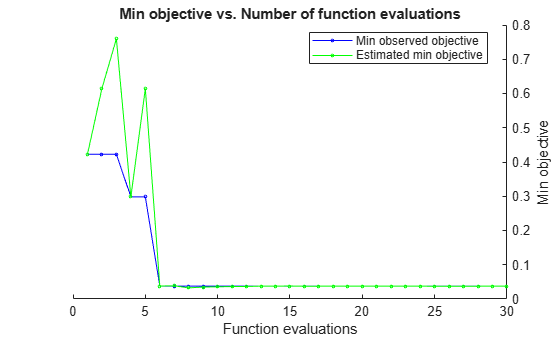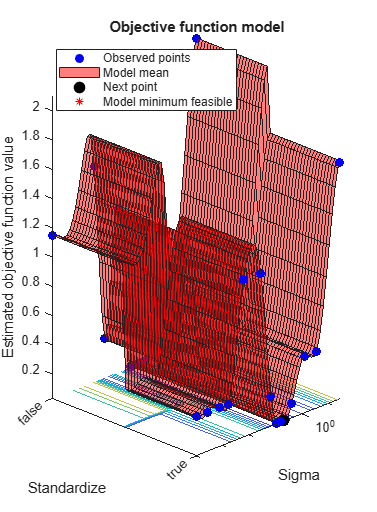```__________________________________________________________ Optimization completed. MaxObjectiveEvaluations of 30 reached. Total function evaluations: 30 Total elapsed time: 92.2108 seconds Total objective function evaluation time: 67.7649 Best observed feasible point: Sigma ______ 0.2367 Observed objective function value = 0.037704 Estimated objective function value = 0.038223 Function evaluation time = 2.528 Best estimated feasible point (according to models): Sigma _______ 0.16159 Estimated objective function value = 0.037881 Estimated function evaluation time = 1.9918 ```

Compare the pre- and post-optimization fits.

```ypred1 = resubPredict(gprMdl1); ypred2 = resubPredict(gprMdl2); figure(); plot(x,y,'r.'); hold on plot(x,ypred1,'b'); plot(x,ypred2,'k','LineWidth',2); xlabel('x'); ylabel('y'); legend({'data','Initial Fit','Optimized Fit'},'Location','Best'); title('Impact of Optimization'); hold off```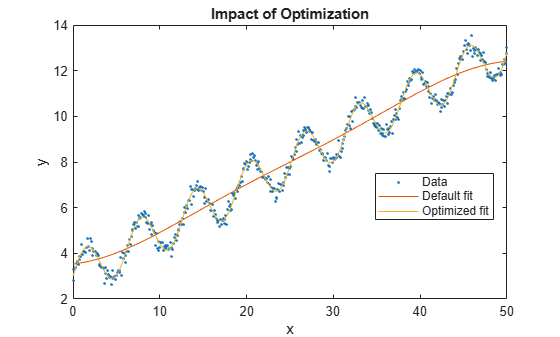This example uses the abalone data , , from the UCI Machine Learning Repository . Download the data and save it in your current folder with the name `abalone.data`.

Store the data into a `table`. Display the first seven rows.

```tbl = readtable('abalone.data','Filetype','text','ReadVariableNames',false); tbl.Properties.VariableNames = {'Sex','Length','Diameter','Height','WWeight','SWeight','VWeight','ShWeight','NoShellRings'}; tbl(1:7,:)```
```ans = Sex Length Diameter Height WWeight SWeight VWeight ShWeight NoShellRings ___ ______ ________ ______ _______ _______ _______ ________ ____________ 'M' 0.455 0.365 0.095 0.514 0.2245 0.101 0.15 15 'M' 0.35 0.265 0.09 0.2255 0.0995 0.0485 0.07 7 'F' 0.53 0.42 0.135 0.677 0.2565 0.1415 0.21 9 'M' 0.44 0.365 0.125 0.516 0.2155 0.114 0.155 10 'I' 0.33 0.255 0.08 0.205 0.0895 0.0395 0.055 7 'I' 0.425 0.3 0.095 0.3515 0.141 0.0775 0.12 8 'F' 0.53 0.415 0.15 0.7775 0.237 0.1415 0.33 20```

The dataset has 4177 observations. The goal is to predict the age of abalone from eight physical measurements. The last variable, number of shell rings shows the age of the abalone. The first predictor is a categorical variable. The last variable in the table is the response variable.

Train a cross-validated GPR model using the 25% of the data for validation.

```rng('default') % For reproducibility cvgprMdl = fitrgp(tbl,'NoShellRings','Standardize',1,'Holdout',0.25); ```

Compute the average loss on folds using models trained on out-of-fold observations.

```kfoldLoss(cvgprMdl) ```
```ans = 4.6409```

Predict the responses for out-of-fold data.

```ypred = kfoldPredict(cvgprMdl); ```

Plot the true responses used for testing and the predictions.

```figure(); plot(ypred(cvgprMdl.Partition.test)); hold on; y = table2array(tbl(:,end)); plot(y(cvgprMdl.Partition.test),'r.'); axis([0 1050 0 30]); xlabel('x') ylabel('y') hold off;```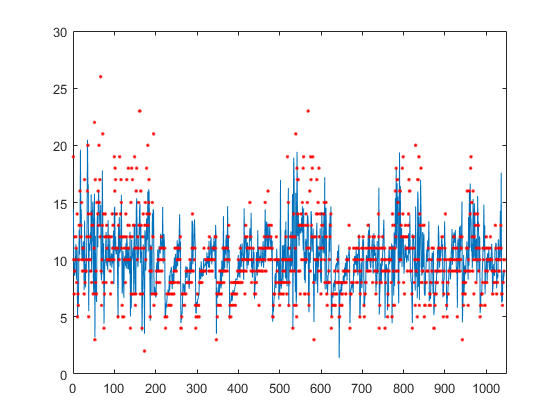Generate the sample data.

```rng(0,'twister'); % For reproducibility n = 1000; x = linspace(-10,10,n)'; y = 1 + x*5e-2 + sin(x)./x + 0.2*randn(n,1);```

Define the squared exponential kernel function as a custom kernel function.

You can compute the squared exponential kernel function as

`$k\left({x}_{i},{x}_{j}|\theta \right)={\sigma }_{f}^{2}\mathrm{exp}\left(-\frac{1}{2}\frac{\left({x}_{i}-{x}_{j}{\right)}^{T}\left({x}_{i}-{x}_{j}\right)}{{\sigma }_{l}^{2}}\right),$`

where ${\sigma }_{f}$ is the signal standard deviation, ${\sigma }_{l}$ is the length scale. Both ${\sigma }_{f}$ and ${\sigma }_{l}$ must be greater than zero. This condition can be enforced by the unconstrained parametrization, ${\sigma }_{l}=\mathrm{exp}\left(\theta \left(1\right)\right)$ and ${\sigma }_{f}=\mathrm{exp}\left(\theta \left(2\right)\right)$, for some unconstrained parametrization vector $\theta$.

Hence, you can define the squared exponential kernel function as a custom kernel function as follows:

`kfcn = @(XN,XM,theta) (exp(theta(2))^2)*exp(-(pdist2(XN,XM).^2)/(2*exp(theta(1))^2));`

Here `pdist2(XN,XM).^2` computes the distance matrix.

Fit a GPR model using the custom kernel function, `kfcn`. Specify the initial values of the kernel parameters (Because you use a custom kernel function, you must provide initial values for the unconstrained parametrization vector, `theta`).

```theta0 = [1.5,0.2]; gprMdl = fitrgp(x,y,'KernelFunction',kfcn,'KernelParameters',theta0);```

`fitrgp` uses analytical derivatives to estimate parameters when using a built-in kernel function, whereas when using a custom kernel function it uses numerical derivatives.

Compute the resubstitution loss for this model.

`L = resubLoss(gprMdl)`
```L = 0.0391 ```

Fit the GPR model using the built-in squared exponential kernel function option. Specify the initial values of the kernel parameters (Because you use the built-in custom kernel function and specifying initial parameter values, you must provide the initial values for the signal standard deviation and length scale(s) directly).

```sigmaL0 = exp(1.5); sigmaF0 = exp(0.2); gprMdl2 = fitrgp(x,y,'KernelFunction','squaredexponential','KernelParameters',[sigmaL0,sigmaF0]);```

Compute the resubstitution loss for this model.

`L2 = resubLoss(gprMdl2)`
```L2 = 0.0391 ```

The two loss values are the same as expected.

Train a GPR model on generated data with many predictors. Specify the initial step size for the LBFGS optimizer.

Set the seed and type of the random number generator for reproducibility of the results.

`rng(0,'twister'); % For reproducibility `

Generate sample data with 300 observations and 3000 predictors, where the response variable depends on the 4th, 7th, and 13th predictors.

```N = 300; P = 3000; X = rand(N,P); y = cos(X(:,7)) + sin(X(:,4).*X(:,13)) + 0.1*randn(N,1);```

Set initial values for the kernel parameters.

```sigmaL0 = sqrt(P)*ones(P,1); % Length scale for predictors sigmaF0 = 1; % Signal standard deviation```

Set initial noise standard deviation to `1`.

`sigmaN0 = 1;`

Specify` 1e-2 `as the termination tolerance for the relative gradient norm.

```opts = statset('fitrgp'); opts.TolFun = 1e-2;```

Fit a GPR model using the initial kernel parameter values, initial noise standard deviation, and an automatic relevance determination (ARD) squared exponential kernel function.

Specify the initial step size as 1 for determining the initial Hessian approximation for an LBFGS optimizer.

```gpr = fitrgp(X,y,'KernelFunction','ardsquaredexponential','Verbose',1, ... 'Optimizer','lbfgs','OptimizerOptions',opts, ... 'KernelParameters',[sigmaL0;sigmaF0],'Sigma',sigmaN0,'InitialStepSize',1);```
```o Parameter estimation: FitMethod = Exact, Optimizer = lbfgs o Solver = LBFGS, HessianHistorySize = 15, LineSearchMethod = weakwolfe |====================================================================================================| | ITER | FUN VALUE | NORM GRAD | NORM STEP | CURV | GAMMA | ALPHA | ACCEPT | |====================================================================================================| | 0 | 3.004966e+02 | 2.569e+02 | 0.000e+00 | | 3.893e-03 | 0.000e+00 | YES | | 1 | 9.525779e+01 | 1.281e+02 | 1.003e+00 | OK | 6.913e-03 | 1.000e+00 | YES | | 2 | 3.972026e+01 | 1.647e+01 | 7.639e-01 | OK | 4.718e-03 | 5.000e-01 | YES | | 3 | 3.893873e+01 | 1.073e+01 | 1.057e-01 | OK | 3.243e-03 | 1.000e+00 | YES | | 4 | 3.859904e+01 | 5.659e+00 | 3.282e-02 | OK | 3.346e-03 | 1.000e+00 | YES | | 5 | 3.748912e+01 | 1.030e+01 | 1.395e-01 | OK | 1.460e-03 | 1.000e+00 | YES | | 6 | 2.028104e+01 | 1.380e+02 | 2.010e+00 | OK | 2.326e-03 | 1.000e+00 | YES | | 7 | 2.001849e+01 | 1.510e+01 | 9.685e-01 | OK | 2.344e-03 | 1.000e+00 | YES | | 8 | -7.706109e+00 | 8.340e+01 | 1.125e+00 | OK | 5.771e-04 | 1.000e+00 | YES | | 9 | -1.786074e+01 | 2.323e+02 | 2.647e+00 | OK | 4.217e-03 | 1.250e-01 | YES | | 10 | -4.058422e+01 | 1.972e+02 | 6.796e-01 | OK | 7.035e-03 | 1.000e+00 | YES | | 11 | -7.850209e+01 | 4.432e+01 | 8.335e-01 | OK | 3.099e-03 | 1.000e+00 | YES | | 12 | -1.312162e+02 | 3.334e+01 | 1.277e+00 | OK | 5.432e-02 | 1.000e+00 | YES | | 13 | -2.005064e+02 | 9.519e+01 | 2.828e+00 | OK | 5.292e-03 | 1.000e+00 | YES | | 14 | -2.070150e+02 | 1.898e+01 | 1.641e+00 | OK | 6.817e-03 | 1.000e+00 | YES | | 15 | -2.108086e+02 | 3.793e+01 | 7.685e-01 | OK | 3.479e-03 | 1.000e+00 | YES | | 16 | -2.122920e+02 | 7.057e+00 | 1.591e-01 | OK | 2.055e-03 | 1.000e+00 | YES | | 17 | -2.125610e+02 | 4.337e+00 | 4.818e-02 | OK | 1.974e-03 | 1.000e+00 | YES | | 18 | -2.130162e+02 | 1.178e+01 | 8.891e-02 | OK | 2.856e-03 | 1.000e+00 | YES | | 19 | -2.139378e+02 | 1.933e+01 | 2.371e-01 | OK | 1.029e-02 | 1.000e+00 | YES | |====================================================================================================| | ITER | FUN VALUE | NORM GRAD | NORM STEP | CURV | GAMMA | ALPHA | ACCEPT | |====================================================================================================| | 20 | -2.151111e+02 | 1.550e+01 | 3.015e-01 | OK | 2.765e-02 | 1.000e+00 | YES | | 21 | -2.173046e+02 | 5.856e+00 | 6.537e-01 | OK | 1.414e-02 | 1.000e+00 | YES | | 22 | -2.201781e+02 | 8.918e+00 | 8.484e-01 | OK | 6.381e-03 | 1.000e+00 | YES | | 23 | -2.288858e+02 | 4.846e+01 | 2.311e+00 | OK | 2.661e-03 | 1.000e+00 | YES | | 24 | -2.392171e+02 | 1.190e+02 | 6.283e+00 | OK | 8.113e-03 | 1.000e+00 | YES | | 25 | -2.511145e+02 | 1.008e+02 | 1.198e+00 | OK | 1.605e-02 | 1.000e+00 | YES | | 26 | -2.742547e+02 | 2.207e+01 | 1.231e+00 | OK | 3.191e-03 | 1.000e+00 | YES | | 27 | -2.849931e+02 | 5.067e+01 | 3.660e+00 | OK | 5.184e-03 | 1.000e+00 | YES | | 28 | -2.899797e+02 | 2.068e+01 | 1.162e+00 | OK | 6.270e-03 | 1.000e+00 | YES | | 29 | -2.916723e+02 | 1.816e+01 | 3.213e-01 | OK | 1.415e-02 | 1.000e+00 | YES | | 30 | -2.947674e+02 | 6.965e+00 | 1.126e+00 | OK | 6.339e-03 | 1.000e+00 | YES | | 31 | -2.962491e+02 | 1.349e+01 | 2.352e-01 | OK | 8.999e-03 | 1.000e+00 | YES | | 32 | -3.004921e+02 | 1.586e+01 | 9.880e-01 | OK | 3.940e-02 | 1.000e+00 | YES | | 33 | -3.118906e+02 | 1.889e+01 | 3.318e+00 | OK | 1.213e-01 | 1.000e+00 | YES | | 34 | -3.189215e+02 | 7.086e+01 | 3.070e+00 | OK | 8.095e-03 | 1.000e+00 | YES | | 35 | -3.245557e+02 | 4.366e+00 | 1.397e+00 | OK | 2.718e-03 | 1.000e+00 | YES | | 36 | -3.254613e+02 | 3.751e+00 | 6.546e-01 | OK | 1.004e-02 | 1.000e+00 | YES | | 37 | -3.262823e+02 | 4.011e+00 | 2.026e-01 | OK | 2.441e-02 | 1.000e+00 | YES | | 38 | -3.325606e+02 | 1.773e+01 | 2.427e+00 | OK | 5.234e-02 | 1.000e+00 | YES | | 39 | -3.350374e+02 | 1.201e+01 | 1.603e+00 | OK | 2.674e-02 | 1.000e+00 | YES | |====================================================================================================| | ITER | FUN VALUE | NORM GRAD | NORM STEP | CURV | GAMMA | ALPHA | ACCEPT | |====================================================================================================| | 40 | -3.379112e+02 | 5.280e+00 | 1.393e+00 | OK | 1.177e-02 | 1.000e+00 | YES | | 41 | -3.389136e+02 | 3.061e+00 | 7.121e-01 | OK | 2.935e-02 | 1.000e+00 | YES | | 42 | -3.401070e+02 | 4.094e+00 | 6.224e-01 | OK | 3.399e-02 | 1.000e+00 | YES | | 43 | -3.436291e+02 | 8.833e+00 | 1.707e+00 | OK | 5.231e-02 | 1.000e+00 | YES | | 44 | -3.456295e+02 | 5.891e+00 | 1.424e+00 | OK | 3.772e-02 | 1.000e+00 | YES | | 45 | -3.460069e+02 | 1.126e+01 | 2.580e+00 | OK | 3.907e-02 | 1.000e+00 | YES | | 46 | -3.481756e+02 | 1.546e+00 | 8.142e-01 | OK | 1.565e-02 | 1.000e+00 | YES | Infinity norm of the final gradient = 1.546e+00 Two norm of the final step = 8.142e-01, TolX = 1.000e-12 Relative infinity norm of the final gradient = 6.016e-03, TolFun = 1.000e-02 EXIT: Local minimum found. o Alpha estimation: PredictMethod = Exact ```

Because the GPR model uses an ARD kernel with many predictors, using an LBFGS approximation to the Hessian is more memory efficient than storing the full Hessian matrix. Also, using the initial step size to determine the initial Hessian approximation may help speed up optimization.

Find the predictor weights by taking the exponential of the negative learned length scales. Normalize the weights.

```sigmaL = gpr.KernelInformation.KernelParameters(1:end-1); % Learned length scales weights = exp(-sigmaL); % Predictor weights weights = weights/sum(weights); % Normalized predictor weights```

Plot the normalized predictor weights.

```figure; semilogx(weights,'ro'); xlabel('Predictor index'); ylabel('Predictor weight');```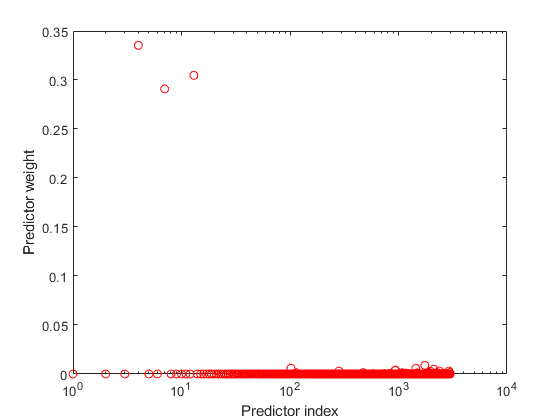The trained GPR model assigns the largest weights to the 4th, 7th, and 13th predictors. The irrelevant predictors have weights close to zero.

## Input Arguments

collapse all

Sample data used to train the model, specified as a `table`. Each row of `Tbl` corresponds to one observation, and each column corresponds to one variable. `Tbl` contains the predictor variables, and optionally it can also contain one column for the response variable. Multicolumn variables and cell arrays other than cell arrays of character vectors are not allowed.

• If `Tbl` contains the response variable, and you want to use all the remaining variables as predictors, then specify the response variable using `ResponseVarName`.

• If `Tbl` contains the response variable, and you want to use only a subset of the predictors in training the model, then specify the response variable and the predictor variables using `formula`.

• If `Tbl` does not contain the response variable, then specify a response variable using `y`. The length of the response variable and the number of rows in `Tbl` must be equal.

For more information on the `table` data type, see `table`.

If your predictor data contains categorical variables, then `fitrgp` creates dummy variables. For details, see `CategoricalPredictors`.

Data Types: `table`

Response variable name, specified as the name of a variable in `Tbl`. You must specify `ResponseVarName` as a character vector or string scalar. For example, if the response variable `y` is stored in `Tbl` (as `Tbl.y`), then specify it as `'y'`. Otherwise, the software treats all the columns of `Tbl`, including `y`, as predictors when training the model.

Data Types: `char` | `string`

Response and predictor variables to use in model training, specified as a character vector or string scalar in the form of `'y~x1+x2+x3'`. In this form, `y` represents the response variable; `x1`, `x2`, `x3` represent the predictor variables to use in training the model.

Use a formula if you want to specify a subset of variables in `Tbl` as predictors to use when training the model. If you specify a formula, then any variables that do not appear in `formula` are not used to train the model.

The variable names in the formula must be both variable names in `Tbl` (`Tbl.Properties.VariableNames`) and valid MATLAB® identifiers. You can verify the variable names in `Tbl` by using the `isvarname` function. If the variable names are not valid, then you can convert them by using the `matlab.lang.makeValidName` function.

The formula does not indicate the form of the `BasisFunction`.

Example: `'PetalLength~PetalWidth+Species'` identifies the variable `PetalLength` as the response variable, and `PetalWidth` and `Species` as the predictor variables.

Data Types: `char` | `string`

Predictor data for the GPR model, specified as an n-by-d matrix. n is the number of observations (rows), and d is the number of predictors (columns).

The length of `y` and the number of rows of `X` must be equal.

To specify the names of the predictors in the order of their appearance in `X`, use the `PredictorNames` name-value pair argument.

Data Types: `double`

Response data for the GPR model, specified as an n-by-1 vector. You can omit `y` if you provide the `Tbl` training data that also includes `y`. In that case, use `ResponseVarName` to identify the response variable or use `formula` to identify the response and predictor variables.

Data Types: `double`

### Name-Value Arguments

Specify optional pairs of arguments as `Name1=Value1,...,NameN=ValueN`, where `Name` is the argument name and `Value` is the corresponding value. Name-value arguments must appear after other arguments, but the order of the pairs does not matter.

Before R2021a, use commas to separate each name and value, and enclose `Name` in quotes.

Example: `'FitMethod','sr','BasisFunction','linear','ActiveSetMethod','sgma','PredictMethod','fic'` trains the GPR model using the subset of regressors approximation method for parameter estimation, uses a linear basis function, uses sparse greedy matrix approximation for active selection, and fully independent conditional approximation method for prediction.

Note

You cannot use any cross-validation name-value argument together with the `'OptimizeHyperparameters'` name-value argument. You can modify the cross-validation for `'OptimizeHyperparameters'` only by using the `'HyperparameterOptimizationOptions'` name-value argument.

Fitting

collapse all

Method to estimate parameters of the GPR model, specified as one of the following.

Fit MethodDescription
`'none'`No estimation, use the initial parameter values as the known parameter values.
`'exact'`Exact Gaussian process regression. Default if n ≤ 2000, where n is the number of observations.
`'sd'`Subset of data points approximation. Default if n > 2000, where n is the number of observations.
`'sr'`Subset of regressors approximation.
`'fic'`Fully independent conditional approximation.

Example: `'FitMethod','fic'`

Explicit basis in the GPR model, specified as one of the following. If n is the number of observations, the basis function adds the term H*β to the model, where H is the basis matrix and β is a p-by-1 vector of basis coefficients.

Explicit BasisBasis Matrix
`'none'`Empty matrix.
`'constant'`

`$H=1$`

(n-by-1 vector of 1s, where n is the number of observations)

`'linear'`

`$H=\left[1,X\right]$`

`'pureQuadratic'`

`$H=\left[1,X,{X}_{2}\right],$`

where

`${X}_{2}=\left[\begin{array}{cccc}{x}_{11}^{2}& {x}_{12}^{2}& \cdots & {x}_{1d}^{2}\\ {x}_{21}^{2}& {x}_{22}^{2}& \cdots & {x}_{2d}^{2}\\ ⋮& ⋮& ⋮& ⋮\\ {x}_{n1}^{2}& {x}_{n2}^{2}& \cdots & {x}_{nd}^{2}\end{array}\right].$`

Function handle

Function handle, `hfcn`, that `fitrgp` calls as:

`$H=hfcn\left(X\right),$`

where `X` is an n-by-d matrix of predictors and H is an n-by-p matrix of basis functions.

Example: `'BasisFunction','pureQuadratic'`

Data Types: `char` | `string` | `function_handle`

Initial value of the coefficients for the explicit basis, specified as a p-by-1 vector, where p is the number of columns in the basis matrix H.

The basis matrix depends on the choice of the explicit basis function as follows (also see `BasisFunction`).

`fitrgp` uses the coefficient initial values as the known coefficient values, only when `FitMethod` is `'none'`.

Data Types: `double`

Initial value for the noise standard deviation of the Gaussian process model, specified as a positive scalar value.

`fitrgp` parameterizes the noise standard deviation as the sum of `SigmaLowerBound` and `exp(η)`, where η is an unconstrained value. Therefore, `Sigma` must be larger than `SigmaLowerBound` by a small tolerance so that the function can initialize η to a finite value. Otherwise, the function resets `Sigma` to a compatible value.

The tolerance is `1e-3` when `ConstantSigma` is `false` (default) and `1e-6` otherwise. If the tolerance is not small enough relative to the scale of the response variable, you can scale up the response variable so that the tolerance value can be considered small for the response variable.

Example: `'Sigma',2`

Data Types: `double`

Constant value of `Sigma` for the noise standard deviation of the Gaussian process model, specified as a logical scalar. When `ConstantSigma` is `true`, `fitrgp` does not optimize the value of `Sigma`, but instead takes the initial value as the value throughout its computations.

Example: `'ConstantSigma',true`

Data Types: `logical`

Lower bound on the noise standard deviation (`Sigma`), specified as a positive scalar value.

`Sigma` must be larger than `SigmaLowerBound` by a small tolerance. For details, see `Sigma`.

Example: `'SigmaLowerBound',0.02`

Data Types: `double`

Categorical predictors list, specified as one of the values in this table.

ValueDescription
Vector of positive integers

Each entry in the vector is an index value indicating that the corresponding predictor is categorical. The index values are between 1 and `p`, where `p` is the number of predictors used to train the model.

If `fitrgp` uses a subset of input variables as predictors, then the function indexes the predictors using only the subset. The `CategoricalPredictors` values do not count the response variable, observation weights variable, or any other variables that the function does not use.

Logical vector

A `true` entry means that the corresponding predictor is categorical. The length of the vector is `p`.

Character matrixEach row of the matrix is the name of a predictor variable. The names must match the entries in `PredictorNames`. Pad the names with extra blanks so each row of the character matrix has the same length.
String array or cell array of character vectorsEach element in the array is the name of a predictor variable. The names must match the entries in `PredictorNames`.
`"all"`All predictors are categorical.

By default, if the predictor data is in a table (`Tbl`), `fitrgp` assumes that a variable is categorical if it is a logical vector, categorical vector, character array, string array, or cell array of character vectors. If the predictor data is a matrix (`X`), `fitrgp` assumes that all predictors are continuous. To identify any other predictors as categorical predictors, specify them by using the `CategoricalPredictors` name-value argument.

For the identified categorical predictors, `fitrgp` creates dummy variables using two different schemes, depending on whether a categorical variable is unordered or ordered. For an unordered categorical variable, `fitrgp` creates one dummy variable for each level of the categorical variable. For an ordered categorical variable, `fitrgp` creates one less dummy variable than the number of categories. For details, see Automatic Creation of Dummy Variables.

Example: `'CategoricalPredictors','all'`

Data Types: `single` | `double` | `logical` | `char` | `string` | `cell`

Indicator to standardize data, specified as a logical value.

If you set `'Standardize',1`, then the software centers and scales each column of the predictor data, by the column mean and standard deviation, respectively. The software does not standardize the data contained in the dummy variable columns that it generates for categorical predictors.

Example: `'Standardize',1`

Example: `'Standardize',true`

Data Types: `logical`

Regularization standard deviation for sparse methods subset of regressors (`'sr'`) and fully independent conditional (`'fic'`), specified as a positive scalar value.

Example: `'Regularization',0.2`

Data Types: `double`

Method for computing the log likelihood and gradient for parameter estimation using subset of regressors (`'sr'`) and fully independent conditional (`'fic'`) approximation methods, specified as one of the following.

• `'qr'` — Use QR factorization based approach, this option provides better accuracy.

• `'v'` — Use V-method-based approach. This option provides faster computation of log likelihood gradients.

Example: `'ComputationMethod','v' `

Kernel (Covariance) Function

collapse all

Form of the covariance function, specified as one of the following.

FunctionDescription
`'exponential'`Exponential kernel.
`'squaredexponential'`Squared exponential kernel.
`'matern32'`Matern kernel with parameter 3/2.
`'matern52'`Matern kernel with parameter 5/2.
`'rationalquadratic'`Rational quadratic kernel.
`'ardexponential'`Exponential kernel with a separate length scale per predictor.
`'ardsquaredexponential'`Squared exponential kernel with a separate length scale per predictor.
`'ardmatern32'`Matern kernel with parameter 3/2 and a separate length scale per predictor.
`'ardmatern52'`Matern kernel with parameter 5/2 and a separate length scale per predictor.
`'ardrationalquadratic'`Rational quadratic kernel with a separate length scale per predictor.
Function handleA function handle that can be called like this:
`Kmn = kfcn(Xm,Xn,theta)`
where `Xm` is an m-by-d matrix, `Xn` is an n-by-d matrix and `Kmn` is an m-by-n matrix of kernel products such that `Kmn`(i,j) is the kernel product between `Xm`(i,:) and `Xn`(j,:).
`theta` is the r-by-1 unconstrained parameter vector for `kfcn`.

For more information on the kernel functions, see Kernel (Covariance) Function Options.

Example: `'KernelFunction','matern32'`

Data Types: `char` | `string` | `function_handle`

Initial values for the kernel parameters, specified as a vector. The size of the vector and the values depend on the form of the covariance function, specified by the `KernelFunction` name-value pair argument.

`'KernelFunction'``'KernelParameters'`
`'exponential'`, `'squaredexponential'`, `'matern32'`, or `'matern52'`2-by-1 vector `phi`, where `phi(1)` contains the length scale and `phi(2)` contains the signal standard deviation.
Default initial value of the length scale parameter is the mean of standard deviations of the predictors, and the signal standard deviation is the standard deviation of the responses divided by square root of 2. That is,
`phi = [mean(std(X));std(y)/sqrt(2)]`
`'rationalquadratic'`3-by-1 vector `phi`, where `phi(1)` contains the length scale, `phi(2)` contains the scale-mixture parameter, and `phi(3)` contains the signal standard deviation.
Default initial value of the length scale parameter is the mean of standard deviations of the predictors, and the signal standard deviation is the standard deviation of the responses divided by square root of 2. Default initial value for the scale-mixture parameter is 1. That is,
`phi = [mean(std(X));1;std(y)/sqrt(2)]`
`'ardexponential'`, `'ardsquaredexponential'`, `'ardmatern32'`, or `'ardmatern52'`(d+1)-by-1 vector `phi`, where `phi(i)` contains the length scale for predictor i, and `phi(d+1)` contains the signal standard deviation. d is the number of predictor variables after dummy variables are created for categorical variables. For details about creating dummy variables, see `CategoricalPredictors`.
Default initial value of the length scale parameters are the standard deviations of the predictors and the signal standard deviation is the standard deviation of the responses divided by square root of 2. That is,
`phi = [std(X)';std(y)/sqrt(2)]`
`'ardrationalquadratic'`(d+2)-by-1 vector `phi`, where `phi(i)` contains the length scale for predictor i, `phi(d+1)` contains the scale-mixture parameter, and `phi(d+2)` contains signal standard deviation.
Default initial value of the length scale parameters are the standard deviations of the predictors and the signal standard deviation is the standard deviation of the responses divided by square root of 2. Default initial value for the scale-mixture parameter is 1. That is,
`phi = [std(X)';1;std(y)/sqrt(2)]`
Function handler-by-1 vector as the initial value of the unconstrained parameter vector `phi` for the custom kernel function `kfcn`.
When `KernelFunction` is a function handle, you must supply initial values for the kernel parameters.

For more information on the kernel functions, see Kernel (Covariance) Function Options.

Example: `'KernelParameters',theta`

Data Types: `double`

Method for computing inter-point distances to evaluate built-in kernel functions, specified as either `'fast'` or `'accurate'`. `fitrgp` computes ${\left(x-y\right)}^{2}$ as ${x}^{2}+{y}^{2}-2*x*y$ when you choose the `fast` option and as ${\left(x-y\right)}^{2}$ when you choose the `accurate` option.

Example: `'DistanceMethod','accurate'`

Active Set Selection

collapse all

Observations in the active set, specified as an m-by-1 vector of integers ranging from 1 to n (mn) or a logical vector of length n with at least one `true` element. n is the total number of observations in the training data.

`fitrgp` uses the observations indicated by `ActiveSet` to train the GPR model. The active set cannot have duplicate elements.

If you supply `ActiveSet`, then:

Data Types: `double` | `logical`

Size of the active set for sparse methods (`'sd'`, `'sr'`, `'fic'`), specified as an integer m, 1 ≤ mn, where n is the number of observations.

Default is min(1000,n) when `FitMethod` is `'sr'` or `'fic'`, and min(2000,n), otherwise.

Example: `'ActiveSetSize',100`

Data Types: `double`

Active set selection method, specified as one of the following.

MethodDescription
`'random'`Random selection
`'sgma'`Sparse greedy matrix approximation
`'entropy'`Differential entropy-based selection
`'likelihood'`Subset of regressors log likelihood-based selection

All active set selection methods (except `'random'`) require the storage of an n-by-m matrix, where m is the size of the active set and n is the number of observations.

Example: `'ActiveSetMethod','entropy'`

Random search set size per greedy inclusion for active set selection, specified as an integer value.

Example: `'RandomSearchSetSize',30`

Data Types: `double`

Relative tolerance for terminating active set selection, specified as a positive scalar value.

Example: `'ToleranceActiveset',0.0002`

Data Types: `double`

Number of repetitions for interleaved active set selection and parameter estimation when `ActiveSetMethod` is not `'random'`, specified as an integer value.

Example: `'NumActiveSetRepeats',5`

Data Types: `double`

Prediction

collapse all

Method used to make predictions from a Gaussian process model given the parameters, specified as one of the following.

MethodDescription
`'exact'`Exact Gaussian process regression method. Default, if n ≤ 10000.
`'bcd'`Block coordinate descent. Default, if n > 10000.
`'sd'`Subset of data points approximation.
`'sr'`Subset of regressors approximation.
`'fic'`Fully independent conditional approximation.

Example: `'PredictMethod','bcd'`

Block size for block coordinate descent method (`'bcd'`), specified as an integer in the range from 1 to n, where n is the number of observations.

Example: `'BlockSizeBCD',1500`

Data Types: `double`

Number of greedy selections for block coordinate descent method (`'bcd'`), specified as an integer in the range from 1 to `BlockSizeBCD`.

Example: `'NumGreedyBCD',150`

Data Types: `double`

Relative tolerance on gradient norm for terminating block coordinate descent method (`'bcd'`) iterations, specified as a positive scalar.

Example: `'ToleranceBCD',0.002`

Data Types: `double`

Absolute tolerance on step size for terminating block coordinate descent method (`'bcd'`) iterations, specified as a positive scalar.

Example: `'StepToleranceBCD',0.002`

Data Types: `double`

Maximum number of block coordinate descent method (`'bcd'`) iterations, specified as an integer value.

Example: `'IterationLimitBCD',10000`

Data Types: `double`

Optimization

collapse all

Optimizer to use for parameter estimation, specified as one of the values in this table.

ValueDescription
`'quasinewton'`Dense, symmetric rank-1-based, quasi-Newton approximation to the Hessian
`'lbfgs'`LBFGS-based quasi-Newton approximation to the Hessian
`'fminsearch'`Unconstrained nonlinear optimization using the simplex search method of Lagarias et al. 
`'fminunc'`Unconstrained nonlinear optimization (requires an Optimization Toolbox™ license)
`'fmincon'`Constrained nonlinear optimization (requires an Optimization Toolbox license)

Example: `'Optimizer','fmincon'`

Options for the optimizer you choose using the `Optimizer` name-value pair argument, specified as a structure or object created by `optimset`, `statset('fitrgp')`, or `optimoptions`.

OptimizerCreate Optimizer Options Using
`'fminsearch'``optimset` (structure)
`'quasinewton'` or `'lbfgs'``statset('fitrgp')` (structure)
`'fminunc'` or `'fmincon'``optimoptions` (object)

The default options depend on the type of optimizer.

Example: `'OptimizerOptions',opt`

Initial step size, specified as a real positive scalar or `'auto'`.

`'InitialStepSize'` is the approximate maximum absolute value of the first optimization step when the optimizer is `'quasinewton'` or `'lbfgs'`. The initial step size can determine the initial Hessian approximation during optimization.

By default, `fitrgp` does not use the initial step size to determine the initial Hessian approximation. To use the initial step size, set a value for the `'InitialStepSize'` name-value pair argument, or specify `'InitialStepSize','auto'` to have `fitrgp` determine a value automatically. For more information on `'auto'`, see Algorithms.

Example: `'InitialStepSize','auto'`

Cross-Validation

collapse all

Indicator for cross-validation, specified as either `'off'` or `'on'`. If it is `'on'`, then `fitrgp` returns a GPR model cross-validated with 10 folds.

You can use one of the `KFold`, `Holdout`, `Leaveout` or `CVPartition` name-value pair arguments to change the default cross-validation settings. You can use only one of these name-value pairs at a time.

As an alternative, you can use the `crossval` method for your model.

Example: `'CrossVal','on'`

Random partition for a stratified k-fold cross-validation, specified as a `cvpartition` object.

Example: `'CVPartition',cvp` uses the random partition defined by `cvp`.

If you specify `CVPartition`, then you cannot specify `Holdout`, `KFold`, or `Leaveout`.

Fraction of the data to use for testing in holdout validation, specified as a scalar value in the range from 0 to 1. If you specify `'Holdout',p`, then the software:
1. Randomly reserves around p*100% of the data as validation data, and trains the model using the rest of the data
2. Stores the compact, trained model in `cvgprMdl.Trained`.

Example: `'Holdout', 0.3` uses 30% of the data for testing and 70% of the data for training.

If you specify `Holdout`, then you cannot specify `CVPartition`, `KFold`, or `Leaveout`.

Data Types: `double`

Number of folds to use in cross-validated GPR model, specified as a positive integer value. `KFold` must be greater than 1. If you specify `'KFold',k` then the software:
1. Randomly partitions the data into k sets.
2. For each set, reserves the set as test data, and trains the model using the other k – 1 sets.
3. Stores the k compact, trained models in the cells of a k-by-1 cell array in `cvgprMdl.Trained`.

Example: `'KFold',5` uses 5 folds in cross-validation. That is, for each fold, uses that fold as test data, and trains the model on the remaining 4 folds.

If you specify `KFold`, then you cannot specify `CVPartition`, `Holdout`, or `Leaveout`.

Data Types: `double`

Indicator for leave-one-out cross-validation, specified as either `'off'` or `'on'`.

If you specify `'Leaveout','on'`, then, for each of the n observations, the software:
1. Reserves the observation as test data, and trains the model using the other n – 1 observations.
2. Stores the compact, trained model in a cell in the n-by-1 cell array `cvgprMdl.Trained`.

Example: `'Leaveout','on'`

If you specify `Leaveout`, then you cannot specify `CVPartition`, `Holdout`, or `KFold`.

Hyperparameter Optimization

collapse all

Parameters to optimize, specified as one of the following:

• `'none'` — Do not optimize.

• `'auto'` — Use `{'Sigma'}`.

• `'all'` — Optimize all eligible parameters, equivalent to`{'BasisFunction','KernelFunction','KernelScale','Sigma','Standardize'}`.

• String array or cell array of eligible parameter names.

• Vector of `optimizableVariable` objects, typically the output of `hyperparameters`.

The optimization attempts to minimize the cross-validation loss (error) for `fitrgp` by varying the parameters. To control the cross-validation type and other aspects of the optimization, use the `HyperparameterOptimizationOptions` name-value pair.

Note

The values of `'OptimizeHyperparameters'` override any values you specify using other name-value arguments. For example, setting `'OptimizeHyperparameters'` to `'auto'` causes `fitrgp` to optimize hyperparameters corresponding to the `'auto'` option and to ignore any specified values for the hyperparameters.

The eligible parameters for `fitrgp` are:

• `BasisFunction``fitrgp` searches among `'constant'`, `'none'`, `'linear'`, and `'pureQuadratic'`.

• `KernelFunction``fitrgp` searches among `'ardexponential'`, `'ardmatern32'`, `'ardmatern52'`, `'ardrationalquadratic'`, `'ardsquaredexponential'`, `'exponential'`, `'matern32'`, `'matern52'`, `'rationalquadratic'`, and `'squaredexponential'`.

• `KernelScale``fitrgp` uses the `KernelParameters` argument to specify the value of the kernel scale parameter, which is held constant during fitting. In this case, all input dimensions are constrained to have the same `KernelScale` value. `fitrgp` searches among real value in the range `[1e-3*MaxPredictorRange,MaxPredictorRange]`, where

`MaxPredictorRange = max(max(X) - min(X))`.

`KernelScale` cannot be optimized for any of the ARD kernels.

• `Sigma``fitrgp` searches among real value in the range `[1e-4, max(1e-3,10*ResponseStd)]`, where

`ResponseStd = std(y)`.

Internally, `fitrgp` sets the `ConstantSigma` name-value pair to `true` so the value of `Sigma` is constant during the fitting.

• `Standardize``fitrgp` searches among `true` and `false`.

Set nondefault parameters by passing a vector of `optimizableVariable` objects that have nondefault values. For example,

```load fisheriris params = hyperparameters('fitrgp',meas,species); params(1).Range = [1e-4,1e6];```

Pass `params` as the value of `OptimizeHyperparameters`.

By default, the iterative display appears at the command line, and plots appear according to the number of hyperparameters in the optimization. For the optimization and plots, the objective function is log(1 + cross-validation loss). To control the iterative display, set the `Verbose` field of the `'HyperparameterOptimizationOptions'` name-value argument. To control the plots, set the `ShowPlots` field of the `'HyperparameterOptimizationOptions'` name-value argument.

For an example, see Optimize GPR Regression.

Example: `'auto'`

Options for optimization, specified as a structure. This argument modifies the effect of the `OptimizeHyperparameters` name-value argument. All fields in the structure are optional.

Field NameValuesDefault
`Optimizer`
• `'bayesopt'` — Use Bayesian optimization. Internally, this setting calls `bayesopt`.

• `'gridsearch'` — Use grid search with `NumGridDivisions` values per dimension.

• `'randomsearch'` — Search at random among `MaxObjectiveEvaluations` points.

`'gridsearch'` searches in a random order, using uniform sampling without replacement from the grid. After optimization, you can get a table in grid order by using the command `sortrows(Mdl.HyperparameterOptimizationResults)`.

`'bayesopt'`
`AcquisitionFunctionName`

• `'expected-improvement-per-second-plus'`

• `'expected-improvement'`

• `'expected-improvement-plus'`

• `'expected-improvement-per-second'`

• `'lower-confidence-bound'`

• `'probability-of-improvement'`

Acquisition functions whose names include `per-second` do not yield reproducible results because the optimization depends on the runtime of the objective function. Acquisition functions whose names include `plus` modify their behavior when they are overexploiting an area. For more details, see Acquisition Function Types.

`'expected-improvement-per-second-plus'`
`MaxObjectiveEvaluations`Maximum number of objective function evaluations.`30` for `'bayesopt'` and `'randomsearch'`, and the entire grid for `'gridsearch'`
`MaxTime`

Time limit, specified as a positive real scalar. The time limit is in seconds, as measured by `tic` and `toc`. The run time can exceed `MaxTime` because `MaxTime` does not interrupt function evaluations.

`Inf`
`NumGridDivisions`For `'gridsearch'`, the number of values in each dimension. The value can be a vector of positive integers giving the number of values for each dimension, or a scalar that applies to all dimensions. This field is ignored for categorical variables.`10`
`ShowPlots`Logical value indicating whether to show plots. If `true`, this field plots the best observed objective function value against the iteration number. If you use Bayesian optimization (`Optimizer` is `'bayesopt'`), then this field also plots the best estimated objective function value. The best observed objective function values and best estimated objective function values correspond to the values in the `BestSoFar (observed)` and ```BestSoFar (estim.)``` columns of the iterative display, respectively. You can find these values in the properties `ObjectiveMinimumTrace` and `EstimatedObjectiveMinimumTrace` of `Mdl.HyperparameterOptimizationResults`. If the problem includes one or two optimization parameters for Bayesian optimization, then `ShowPlots` also plots a model of the objective function against the parameters.`true`
`SaveIntermediateResults`Logical value indicating whether to save results when `Optimizer` is `'bayesopt'`. If `true`, this field overwrites a workspace variable named `'BayesoptResults'` at each iteration. The variable is a `BayesianOptimization` object.`false`
`Verbose`

Display at the command line:

• `0` — No iterative display

• `1` — Iterative display

• `2` — Iterative display with extra information

For details, see the `bayesopt` `Verbose` name-value argument and the example Optimize Classifier Fit Using Bayesian Optimization.

`1`
`UseParallel`Logical value indicating whether to run Bayesian optimization in parallel, which requires Parallel Computing Toolbox™. Due to the nonreproducibility of parallel timing, parallel Bayesian optimization does not necessarily yield reproducible results. For details, see Parallel Bayesian Optimization.`false`
`Repartition`

Logical value indicating whether to repartition the cross-validation at every iteration. If this field is `false`, the optimizer uses a single partition for the optimization.

The setting `true` usually gives the most robust results because it takes partitioning noise into account. However, for good results, `true` requires at least twice as many function evaluations.

`false`
Use no more than one of the following three options.
`CVPartition`A `cvpartition` object, as created by `cvpartition``'Kfold',5` if you do not specify a cross-validation field
`Holdout`A scalar in the range `(0,1)` representing the holdout fraction
`Kfold`An integer greater than 1

Example: `'HyperparameterOptimizationOptions',struct('MaxObjectiveEvaluations',60)`

Data Types: `struct`

Other

collapse all

Predictor variable names, specified as a string array of unique names or a cell array of unique character vectors. The functionality of `'PredictorNames'` depends on the way you supply the training data.

• If you supply `X` and `y`, then you can use `'PredictorNames'` to give the predictor variables in `X` names.

• The order of the names in `PredictorNames` must correspond to the column order of `X`. That is, `PredictorNames{1}` is the name of `X(:,1)`, `PredictorNames{2}` is the name of `X(:,2)`, and so on. Also, `size(X,2)` and `numel(PredictorNames)` must be equal.

• By default, `PredictorNames` is `{'x1','x2',...}`.

• If you supply `Tbl`, then you can use `'PredictorNames'` to choose which predictor variables to use in training. That is, `fitrgp` uses the predictor variables in `PredictorNames` and the response only in training.

• `PredictorNames` must be a subset of `Tbl.Properties.VariableNames` and cannot include the name of the response variable.

• By default, `PredictorNames` contains the names of all predictor variables.

• It good practice to specify the predictors for training using one of `'PredictorNames'` or `formula` only.

Example: `'PredictorNames',{'PedalLength','PedalWidth'}`

Data Types: `string` | `cell`

Response variable name, specified as a character vector or string scalar.

Example: `"ResponseName","response"`

Data Types: `char` | `string`

Verbosity level, specified as one of the following.

• 0 — `fitrgp` suppresses diagnostic messages related to active set selection and block coordinate descent but displays the messages related to parameter estimation, depending on the value of `'Display'` in `OptimizerOptions`.

• 1 — `fitrgp` displays the iterative diagnostic messages related to parameter estimation, active set selection, and block coordinate descent.

Example: `'Verbose',1`

Cache size in megabytes (MB), specified as a positive scalar. Cache size is the extra memory that is available in addition to that required for fitting and active set selection. `fitrgp` uses `CacheSize` to:

• Decide whether interpoint distances should be cached when estimating parameters.

• Decide how matrix vector products should be computed for block coordinate descent method and for making predictions.

Example: `'CacheSize',2000`

Data Types: `double`

## Output Arguments

collapse all

Gaussian process regression model, returned as a `RegressionGP` or `RegressionPartitionedGP` object.

• If you cross-validate, that is, if you use one of the `'Crossval'`, `'KFold'`, `'Holdout'`, `'Leaveout'`, or `'CVPartition'` name-value arguments, then `gprMdl` is a `RegressionPartitionedGP` object. You can use `kfoldPredict` to predict responses for observations that `fitrgp` holds out during training. `kfoldPredict` predicts a response for every observation by using the model trained without that observation. You cannot compute the prediction intervals for a cross-validated model.

• If you do not cross-validate, then `gprMdl` is a `RegressionGP` object. You can use `predict` to predict responses for new observations, and use `resubPredict` to predict responses for training observations. You can also compute the prediction intervals by using `predict` and `resubPredict`.

collapse all

### Active Set Selection and Parameter Estimation

For subset of data, subset of regressors, or fully independent conditional approximation fitting methods (`FitMethod` equal to `'sd'`, `'sr'`, or `'fic'`), if you do not provide the active set (or inducing input set), `fitrgp` selects the active set and computes the parameter estimates in a series of iterations.

In the first iteration, the software uses the initial parameter values in vector η0 = [β0,σ0,θ0] to select an active set A1. It maximizes the GPR marginal log likelihood or its approximation using η0 as the initial values and A1 to compute the new parameter estimates η1. Next, it computes the new log likelihood L1 using η1 and A1.

In the second iteration, the software selects the active set A2 using the parameter values in η1. Then, using η1 as the initial values and A2, it maximizes the GPR marginal log likelihood or its approximation and estimates the new parameter values η2. Then using η2 and A2, computes the new log likelihood value L2.

The following table summarizes the iterations and what is computed at each iteration.

Iteration NumberActive SetParameter VectorLog Likelihood
1A1η1L1
2A2η2L2
3A3η3L3

The software iterates similarly for a specified number of repetitions. You can specify the number of replications for active set selection using the `NumActiveSetRepeats` name-value pair argument.

## Tips

• `fitrgp` accepts any combination of fitting, prediction, and active set selection methods. In some cases it might not be possible to compute the standard deviations of the predicted responses, hence the prediction intervals. See `predict`. And in some cases, using the exact method might be expensive due to the size of the training data.

• The `PredictorNames` property stores one element for each of the original predictor variable names. For example, if there are three predictors, one of which is a categorical variable with three levels, `PredictorNames` is a 1-by-3 cell array of character vectors.

• The `ExpandedPredictorNames` property stores one element for each of the predictor variables, including the dummy variables. For example, if there are three predictors, one of which is a categorical variable with three levels, then `ExpandedPredictorNames` is a 1-by-5 cell array of character vectors.

• Similarly, the `Beta` property stores one beta coefficient for each predictor, including the dummy variables.

• The `X` property stores the training data as originally input. It does not include the dummy variables.

• The default approach to initializing the Hessian approximation in `fitrgp` can be slow when you have a GPR model with many kernel parameters, such as when using an ARD kernel with many predictors. In this case, consider specifying `'auto'` or a value for the initial step size.

You can set `'Verbose',1` for display of iterative diagnostic messages, and begin training a GPR model using an LBFGS or quasi-Newton optimizer with the default `fitrgp` optimization. If the iterative diagnostic messages are not displayed after a few seconds, it is possible that initialization of the Hessian approximation is taking too long. In this case, consider restarting training and using the initial step size to speed up optimization.

• After training a model, you can generate C/C++ code that predicts responses for new data. Generating C/C++ code requires MATLAB Coder™. For details, see Introduction to Code Generation..

## Algorithms

• Fitting a GPR model involves estimating the following model parameters from the data:

• Covariance function $k\left({x}_{i},{x}_{j}|\theta \right)$ parameterized in terms of kernel parameters in vector $\theta$ (see Kernel (Covariance) Function Options)

• Noise variance, ${\sigma }^{2}$

• Coefficient vector of fixed basis functions, $\beta$

The value of the `'KernelParameters'` name-value pair argument is a vector that consists of initial values for the signal standard deviation ${\sigma }_{f}$ and the characteristic length scales ${\sigma }_{l}$. The `fitrgp` function uses these values to determine the kernel parameters. Similarly, the `'Sigma'` name-value pair argument contains the initial value for the noise standard deviation $\sigma$.

• During optimization, `fitrgp` creates a vector of unconstrained initial parameter values ${\eta }_{0}$ by using the initial values for the noise standard deviation and the kernel parameters.

• `fitrgp` analytically determines the explicit basis coefficients $\beta$, specified by the `'Beta'` name-value pair argument, from estimated values of $\theta$ and ${\sigma }^{2}$. Therefore, $\beta$ does not appear in the ${\eta }_{0}$ vector when `fitrgp` initializes numerical optimization.

Note

If you specify no estimation of parameters for the GPR model, `fitrgp` uses the value of the `'Beta'` name-value pair argument and other initial parameter values as the known GPR parameter values (see `Beta`). In all other cases, the value of the `'Beta'` argument is optimized analytically from the objective function.

• The quasi-Newton optimizer uses a trust-region method with a dense, symmetric rank-1-based (SR1), quasi-Newton approximation to the Hessian, while the LBFGS optimizer uses a standard line-search method with a limited-memory Broyden-Fletcher-Goldfarb-Shanno (LBFGS) quasi-Newton approximation to the Hessian. See Nocedal and Wright .

• If you set the `'InitialStepSize'` name-value pair argument to `'auto'`, `fitrgp` determines the initial step size, ${‖{s}_{0}‖}_{\infty }$, by using ${‖{s}_{0}‖}_{\infty }=0.5{‖{\eta }_{0}‖}_{\infty }+0.1$.

${s}_{0}$ is the initial step vector, and ${\eta }_{0}$ is the vector of unconstrained initial parameter values.

• During optimization, `fitrgp` uses the initial step size, ${‖{s}_{0}‖}_{\infty }$, as follows:

If you use `'Optimizer','quasinewton'` with the initial step size, then the initial Hessian approximation is $\frac{{‖{g}_{0}‖}_{\infty }}{{‖{s}_{0}‖}_{\infty }}I$.

If you use `'Optimizer','lbfgs'` with the initial step size, then the initial inverse-Hessian approximation is $\frac{{‖{s}_{0}‖}_{\infty }}{{‖{g}_{0}‖}_{\infty }}I$.

${g}_{0}$ is the initial gradient vector, and $I$ is the identity matrix.

 Nash, W.J., T. L. Sellers, S. R. Talbot, A. J. Cawthorn, and W. B. Ford. "The Population Biology of Abalone (Haliotis species) in Tasmania. I. Blacklip Abalone (H. rubra) from the North Coast and Islands of Bass Strait." Sea Fisheries Division, Technical Report No. 48, 1994.

 Waugh, S. "Extending and Benchmarking Cascade-Correlation: Extensions to the Cascade-Correlation Architecture and Benchmarking of Feed-forward Supervised Artificial Neural Networks." University of Tasmania Department of Computer Science thesis, 1995.

 Lichman, M. UCI Machine Learning Repository, Irvine, CA: University of California, School of Information and Computer Science, 2013. http://archive.ics.uci.edu/ml.

 Rasmussen, C. E. and C. K. I. Williams. Gaussian Processes for Machine Learning. MIT Press. Cambridge, Massachusetts, 2006.

 Lagarias, J. C., J. A. Reeds, M. H. Wright, and P. E. Wright. "Convergence Properties of the Nelder-Mead Simplex Method in Low Dimensions." SIAM Journal of Optimization. Vol. 9, Number 1, 1998, pp. 112–147.

 Nocedal, J. and S. J. Wright. Numerical Optimization, Second Edition. Springer Series in Operations Research, Springer Verlag, 2006.